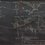# Largest squarefree divisor

It is known that $\displaystyle \lim_{k \to \infty} \left( \sum_i x_i^k \right)^{1/k} = \max_i x_i$ for $x_i \in \mathbb{R}^+$. So, to extract the squarefree divisors of $n$ only, we make use of the Möbius function and take the following sum:

$\sum_{d|n} \mu^2(d)d^k = n^k \sum_{d|n} \mu^2(d)\left( \frac{n}{d} \right)^{-k} = n^k (\mu^2 * \text{Id}_{-k})(n)$

Taking the $k$th root and letting $k \to \infty$:

$\max_{d|n} \mu^2(d)d = \lim_{k \to \infty} \left( n^k (\mu^2 * \text{Id}_{-k})(n) \right)^{1/k} = n \lim_{k \to \infty} (\mu^2 * \text{Id}_{-k})(n)^{1/k}$

Note:

$*$ is the Dirichlet convolution, a binary operator defined on functions $\mathbb{N} \longrightarrow \mathbb{C}$ such that $\displaystyle (f*g)(n) = \sum_{d|n} f(d)g(\frac{n}{d})$.

$\text{Id}_{k}(n) = n^k$ for $n \in \mathbb{N}$, $k \in \mathbb{C}$.Note by Jake Lai
5 years, 10 months ago

This discussion board is a place to discuss our Daily Challenges and the math and science related to those challenges. Explanations are more than just a solution — they should explain the steps and thinking strategies that you used to obtain the solution. Comments should further the discussion of math and science.

When posting on Brilliant:

• Use the emojis to react to an explanation, whether you're congratulating a job well done , or just really confused .
• Ask specific questions about the challenge or the steps in somebody's explanation. Well-posed questions can add a lot to the discussion, but posting "I don't understand!" doesn't help anyone.
• Try to contribute something new to the discussion, whether it is an extension, generalization or other idea related to the challenge.

MarkdownAppears as
*italics* or _italics_ italics
**bold** or __bold__ bold
- bulleted- list
• bulleted
• list
1. numbered2. list
1. numbered
2. list
Note: you must add a full line of space before and after lists for them to show up correctly
paragraph 1paragraph 2

paragraph 1

paragraph 2

[example link](https://brilliant.org)example link
> This is a quote
This is a quote
    # I indented these lines
# 4 spaces, and now they show
# up as a code block.

print "hello world"
# I indented these lines
# 4 spaces, and now they show
# up as a code block.

print "hello world"
MathAppears as
Remember to wrap math in $$ ... $$ or $ ... $ to ensure proper formatting.
2 \times 3 $2 \times 3$
2^{34} $2^{34}$
a_{i-1} $a_{i-1}$
\frac{2}{3} $\frac{2}{3}$
\sqrt{2} $\sqrt{2}$
\sum_{i=1}^3 $\sum_{i=1}^3$
\sin \theta $\sin \theta$
\boxed{123} $\boxed{123}$

Sort by:

Mind if you explain the first step? The

$\displaystyle \lim_{k \to \infty} \left( \sum_i x_i^k \right)^{1/k} = \max_i x_i$

?

- 5 years, 10 months ago

It just means $\displaystyle \lim_{k \to \infty} \left( \sum_{i=1}^n x_i^k \right)^{1/k} = \max_{i=1,2,\ldots ,n} x_i = \max \{ x_1,x_2, \ldots ,x_n \}$.

- 5 years, 10 months ago

No, as to why it is so. Also mind if you also define what $\text{Id}$ means? Thanks

- 5 years, 10 months ago

This is a clean, nice proof. Also I've added clarifications to the note.

- 5 years, 10 months ago